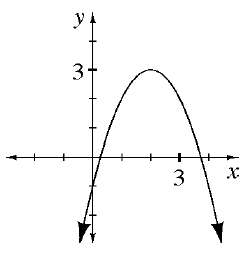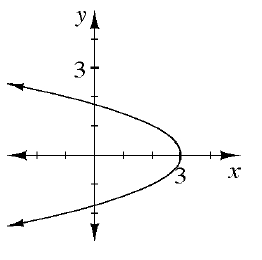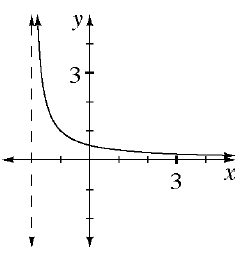### Home > INT3 > Chapter 2 > Lesson 2.2.1 > Problem2-36

2-36.

Match each graph below with its domain. Then state the range of each graph.

1. D: all real numbers

1. D: $x>-2$

1. D: $x\le3$

1)$1$

2)$3$

3)$2$